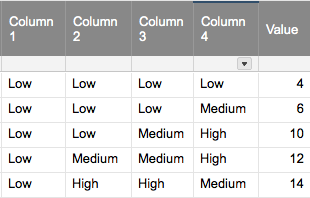or Explore Discussions

# Formula for text columns convert to sum?

12/04/18 Edited 12/09/19

Hello,

I'm trying to help build a sort of prioritization scorecard into a sheet. We have 4 columns, each with possible values of (example) "A", "B" or "C", and would like to have another column that sums each based on assigning a number value to each dropdown option.

So for example, the columns and their possible dropdowns:

Column 1 - A, B, C

Column 2 - A, B, C

Column 3 - A, B, C

Column 4 - A, B, C

Where A = 1, B = 2, C = 3

IF Column 1 = A, Column 2 = B, Column 3 = A, and Column 4 = C, the total value presented would be 7 (1 + 2 + 1 + 3).

I'm aware we could add 4 additional columns to present the numerical value attached to each, then a 5th column with the sum of those. However, I'll be frank: this is a monster sheet and adding even more columns when I'm hoping for a "simple" formula makes me want to cry.

Any suggestions? From what I'm reading on other forum posts I *think* this is impossible but would really appreciate a confirmation. Thanks!

Tags:

• Are the 4 columns adjacent? You could add multiple COUNTIF statements together:

=COUNTIF([Column 1]@row:[Column 4]@row, "A") + (COUNTIF([Column 1]@row:[Column 4]@row, "B") * 2) + (COUNTIF([Column 1]@row:[Column 4]@row, "C") * 3)

• I suppose the columns wouldn’t even need to be adjacent as long as the columns in between don’t contain just A,B, or C.

• Hi Brian, thanks for your help but I'm thinking we crossed wires somewhere.

Let me flesh out the information a bit. So here's what the columns are actually representing:

Scorecard criteria 1:

- Low

- Medium

- High

Scorecard criteria 2:

- Low

- Medium

- High

Scorecard criteria 3:

- Low

- Medium

- High

Scorecard criteria 4:

- Low

- Medium

- High

For all columns:

Low = 1

- Medium = 3

- High = 5

So, as an example of a real-world use:

Scorecard criteria 1: Low (((1)))

Scorecard criteria 2: High (((5)))

Scorecard criteria 3: High (((5)))

Scorecard criteria 4: Medium (((3)))

The formula in a fifth column would return a total of "14" (since there was one "Low", two "High"s, and one "Medium").

Does that make more sense?

• It sounds like this formula should work for you, unless there’s something I’m missing:

=COUNTIF([Column 1]@row:[Column 4]@row, "Low") + (COUNTIF([Column 1]@row:[Column 4]@row, "Medium") * 3) + (COUNTIF([Column 1]@row:[Column 4]@row, "High") * 5)

This formula assumes that your criteria columns are named Column 1, Column 2, Column 3, and Column 4. The formula searches all the cells in a row between Column 1 and Column 4 and counts the instances of Low, Medium, High, then multiplies those counts by 1, 3, and 5 respectively and adds them together.• Way to go Brian!! That's exactly what we were looking for. Thank you so much for your help, much appreciated!!

• Sorry Brian, in my excitement at your perfect answer I forgot to ask one more add-on. This isn't something we're implementing on Day 1 so it's okay if we can't figure it out right now. But after the countif, is there a way of populating (instead of the numerical value) a word? So, for example, if the overall score comes back as between 0 and 10, the value shown in the cell should be something like "Low importance". If it's between 10-20, it should read "Medium importance", etc. What's the framework for adding something like that if at all? Many thanks again.

• Sure. You could use the formula in a nested IF formula:

=IF(COUNTIF([Column 1]@row:[Column 4]@row, "Low") + (COUNTIF([Column 1]@row:[Column 4]@row, "Medium") * 3) + (COUNTIF([Column 1]@row:[Column 4]@row, "High") * 5) >= 20, "High Importance", IF(COUNTIF([Column 1]@row:[Column 4]@row, "Low") + (COUNTIF([Column 1]@row:[Column 4]@row, "Medium") * 3) + (COUNTIF([Column 1]@row:[Column 4]@row, "High") * 5) >= 10, "Medium Importance", IF(COUNTIF([Column 1]@row:[Column 4]@row, "Low") + (COUNTIF([Column 1]@row:[Column 4]@row, "Medium") * 3) + (COUNTIF([Column 1]@row:[Column 4]@row, "High") * 5) > 0, "Low Importance")))

In this version, it returns “High Importance” if the count is 20+, "Medium Importance” if 10-19, or  "Low Importance” for 1-9.